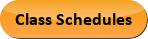Oct 05, 2022
2022-2023 College Catalog
 Select a Catalog 2022-2023 College Catalog 2021-2022 College Catalog [ARCHIVED CATALOG] 2020-2021 College Catalog [ARCHIVED CATALOG] 2019-2020 College Catalog [ARCHIVED CATALOG]
 HELP 2022-2023 College Catalog Print-Friendly Page (opens a new window)

# MAT 241 - Calculus III [SUN# MAT 2241]

4 Credits, 4 Contact Hours
4 lecture periods 0 lab periods

Continuation of MAT 231 . Includes vectors in two and three dimensions, vector-valued functions, differentiation and integration of multivariable functions, and calculus of vector fields.

Prerequisite(s): Within the last three years: MAT 231  with a grade of C or better.
Gen-Ed: Meets AGEC - MATH; Meets CTE - M&S.Course Learning Outcomes
1.   Use vector operations to calculate equations of planes and vector equations of lines.

2.   Use partial derivatives to analyze rates of change of multivariable functions in a variety of contexts.

3.   Evaluate double and triple integrals of multivariable functions in a variety of coordinate systems.

4.   Evaluate line and surface integrals in vector fields using a variety of theorems and techniques.

Performance Objectives:
1.   Use a Cartesian coordinate system in 3-dimensional space; perform vector operations including the dot and cross products; and find the orthogonal projection onto a vector.

2.   Determine equations of lines and planes in space, and identify and classify quadric surfaces.

3.   Evaluate limits, derivatives and integrals of vector-valued functions; analyze motion along a curve; and calculate the unit tangent vector, the unit normal vector, and the curvature.

4.   Evaluate limits, determine continuity, and calculate partial derivatives of multivariable functions; apply the chain rule and use implicit differentiation; calculate directional derivatives and gradient vectors; find equations of tangent planes; determine extrema and saddle points; and use Lagrange multipliers to find constrained maximum and minimum.

5.   Evaluate double integrals in rectangular and polar coordinates; convert between rectangular, cylindrical, and spherical coordinates; evaluate triple integrals in rectangular, cylindrical, and spherical coordinates; and use double and triple integrals to calculate volumes.

6.   Determine if a vector field is conservative and find a potential function; evaluate line integrals of real-valued functions and vector fields; evaluate surface integrals of real-valued functions and vector fields; and use Green’s Theorem, Stokes’ Theorem, and the Divergence Theorem to evaluate line integrals and surface integrals.

Outline:
1. Vectors and Analytic Geometry in the Plane and in Space
1. Vectors in the plane and in space
2. Dot product and cross product
3. Orthogonal projections
2. Lines, Planes, and Surfaces
1. Lines and planes in space
3. Vector Valued Functions
1. Graph of a vector valued function
2. Parametrized curves
3. Arc length
4. Unit tangent vector, unit normal vector, and curvature
5. Projectile motion
4. Functions of Two or More Variables
1. Domain
2. Limits and continuity
3. Partial derivatives
4. Differentiability
5. Chain rule
6. Implicit differentiation
7. Linearization and differentials
8. Directional derivatives, gradient vectors, and tangent planes
9. Local extrema and saddle points
10. Absolute extrema
11. Lagrange multipliers
5. Multiple Integrals
1. Double integrals in rectangular and polar coordinates
2. Cylindrical and spherical coordinates
3. Triple integrals in rectangular, cylindrical, and spherical coordinates
4. Applications
6. Calculus of Vector Fields
1. Vector fields
2. Line integrals
3. Path independence, potential functions, and conservative vector fields
4. Parametrized surfaces
5. Surface area and surface integrals
6. Divergence and curl
7. Green’s Theorem
8. Divergence Theorem and Stokes’ Theorem
9. Applications

Effective Term:
Spring 2020